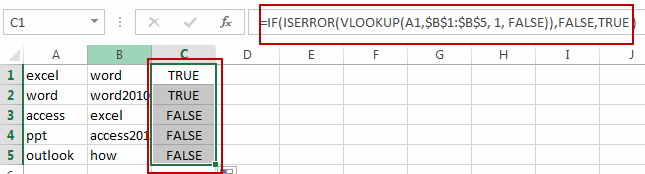# Check If Value in a Column exists in Another Column in Excel

This post will guide you how to check if one value in a column exists in another column in Excel. How do I check if value in column A exists in Column B with a formula in Excel. How to determine if values exists in another column in Excel.

## Check If Value in a Column exists in Another Column

Assuming that you have a list of data in two columns in range A1:B5, and you want to check if one value in column A exists in column B, if True, then return true, otherwise, returns False. How to achieve it. You can create a new formula based on the IF function, the ISERROR function and the VLOOKUP function. Like this:

=IF(ISERROR(VLOOKUP(A1,\$B\$1:\$B\$5, 1, FALSE)),FALSE,TRUE )

Type this formula into cell C1, and press Enter key in your keyboard, and then drag the autofill handle over other cells to apply this formula to check other cells in Column A.### Related Functions

• Excel IF function
The Excel IF function perform a logical test to return one value if the condition is TRUE and return another value if the condition is FALSE. The IF function is a build-in function in Microsoft Excel and it is categorized as a Logical Function.The syntax of the IF function is as below:= IF (condition, [true_value], [false_value])….
• Excel VLOOKUP function
The Excel VLOOKUP function lookup a value in the first column of the table and return the value in the same row based on index_num position.The syntax of the VLOOKUP function is as below:= VLOOKUP (lookup_value, table_array, column_index_num,[range_lookup])….
• Excel ISERROR function
The Excel ISERROR function used to check for any error type that excel generates and it returns TRUE for any error type, and the ISERR function also can be checked for error values except #N/A error, it returns TRUE while the error is #N/A. The syntax of the ISERROR function is as below:= ISERROR (value)….